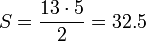# Category Archives: ALAT PERAGA

## MISSING TRIANGLE

The key to the puzzle is the fact that neither of the 13×5 “triangles” is truly a triangle, because what would be the hypotenuse is bent. In other words, the hypotenuse does not maintain a consistent slope, even though it may appear that way to the human eye. A true 13 × 5 triangle cannot be created from the given component parts.The four figures (the yellow, red, blue and green shapes) total 32 units of area, but the triangles are 13 wide and 5 tall, so it seems, that the area should beunits. But the blue triangle has a ratio of 5:2 (=2.5:1), while the red triangle has the ratio 8:3 (≈2.667:1), and these are not the same ratio. So the apparent combined hypotenuse in each figure is actually bent.

The amount of bending is around 1/28th of a unit (1.245364267°), which is difficult to see on the diagram of this puzzle. Note the grid point where the red and blue hypotenuses meet, and compare it to the same point on the other figure; the edge is slightly over or under the mark. Overlaying the hypotenuses from both figures results in a very thin parallelogram with the area of exactly one grid square, the same area “missing” from the second figure.

## MISSING SQUARE PUZZLEA different puzzle of the same kind (depicted in the animation) uses four equal quadrilaterals and a small square, which form a larger square. When the quadrilaterals are rotated about their centers they fill the space of the small square, although the total area of the figure seems unchanged. The apparent paradox is explained by the fact that the side of the new large square is a little smaller than the original one. If a is the side of the large square and θ is the angle between two opposing sides in each quadrilateral, then the quotient between the two areas is given by sec2θ − 1. For θ = 5°, this is approximately 1.00765, which corresponds to a difference of about 0.8%.

## PERMAINAN TRANSFORMASI

###TUJUAN

Tujuan permainan ini adalah untuk mempermudah dan membuat siswa terampil melakukan trasformasi serta lebih memahami materi transformasi.

### SASARAN

Permainan ini digunakan untuk siswa SMA.